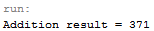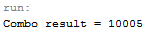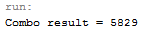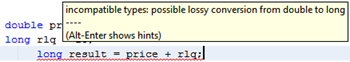# Addition in Java: Code, Method & Examples

Instructor: Martin Gibbs

Martin has 16 years experience in Human Resources Information Systems and has a PhD in Information Technology Management. He is an adjunct professor of computer science and computer programming.

Java provides support for a wide range of arithmetic operations, from the simple to the extremely complex. This lesson will provide an overview of addition operations in Java, providing methods and code samples.

## Math Operators in Java

If you became a programmer to escape math, we have bad news for you. Math is everywhere in programming. The tools learned in math instruction are all available within Java. As an overview, Java provides the following basic arithmetic operations:

Operator Use
- Subtract
/ Divide
* Multiply
% Modulo - Remainder

## Addition in Java

Let's start with the basics and focus on addition. Using the plus operator (+) we can perform some straightforward operations. Let's start with a few integer variables and add them together.

`int a = 12;int b = 17;int c = 342;int d = a + b + c;System.out.println("Addition result = " + d);`

When the program runs, the following output is displayed:Now that we have the basics, we can begin combining.

## Combining Statements

Most complex algorithms are rarely confined to one operation. Java allows you to create any type of mathematical operation, including addition, subtraction, multiplication, and division. Just remember that the order of operations applies to these operations! For example, multiplication and division statements are calculated before addition and subtraction. And, values within parentheses are calculated before items outside parentheses.

In order to check that your code is correct, do some desk-checking and run sample numbers through the code manually. Then run the Java program to make sure it is all in sync. Let's keep some of the same values from before, but we'll update the d variable and add another one (e). This time we'll add in (pun intended) some multiplication.

`int a = 12;int b = 17;int c = 342;int d = 3;int e = (a + b) * (c + d);System.out.println("Combo result = " + e);`

There is only one multiplication operation, but two nested addition operations. We will add a + b (12 + 17) and get 29. Next, c + d (342 + 3) = 345. Finally, 29 * 345 = 10,005. After running the program, we see that our calculations are correct.What happens if we shift the parenthesis around?

`int a = 12;int b = 17;int c = 342;int d = 3;int e = a + (b * c) + d;System.out.println("Combo result = " + e);`

Now we have to do the multiplication first; b * c = 5814. Then add a and d to it, which should give us 5829. Is this right?## Mixed Types

What happens if you try to add a long and a double together? The good news is that Java allows this, for the most part. The following code adds a double and a long (you could use an int here) and displays the output.

`double price = 35.78;long rlq = 15;System.out.println(price + rlq);`

When the code runs, it will display 50.78. However, be careful if you assign a variable to hold this result. That variable should be double, not int or long! If you try to create a variable that can't hold the result, the developer tool or compiler will display a message like the following:To unlock this lesson you must be a Study.com Member.

### Register to view this lesson

Are you a student or a teacher?

### Unlock Your Education

#### See for yourself why 30 million people use Study.com

##### Become a Study.com member and start learning now.
Back
What teachers are saying about Study.com

### Earning College Credit

Did you know… We have over 160 college courses that prepare you to earn credit by exam that is accepted by over 1,500 colleges and universities. You can test out of the first two years of college and save thousands off your degree. Anyone can earn credit-by-exam regardless of age or education level.